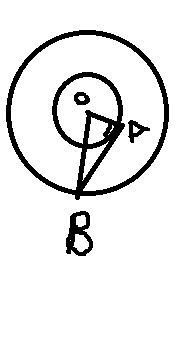# What is the length of the tangent drawn from any point of the circle x2+y2+2gx+2fy+c=0 to the circle x2+y2+2gx+2fy+d=0 ?

11 years ago

Hi Sohan,

Let (a,b) be any point on the circle, from where the tangent is drawn to the other circle.

(a,b) would satisfy the eqn of the first circle.

Let of tangent from any point (a,b) to the second ciscle is √(S1)

where S1 is obtained by substituting (a,b) in the second equation.

So S1 = a2+b2+2ga+2fb+c.

But a2+b2+2ga+2fb+ d = 0.

So S1 = c-d.

So length of tangent = √(c-d).

Best Regards,

11 years ago

the two eqn clearly represent concentric circles as they have centrs at (-g,-f) .
Now
x2+y2+2gx+2fy+c=0   ....... 1
x2+y2+2gx+2fy+d=0  ........2

Eqn 1 repesent circle wid larger radius n the latter(2) represent smaller radius.
Both the cirHere AB is required tangent and OA is radius of smaller circle represented by eqn 2 whereas OB is radius of larger circle represented by eqn 1

NOW
OA=
√g2+f2-d
OB=√g2+f2-c

Applying pythagoras theorom in traingle OAB we have ,

AB2=OB2-OA2
= g2+f2-c -(g2+f2-d)
= d-c
AB =√d-c